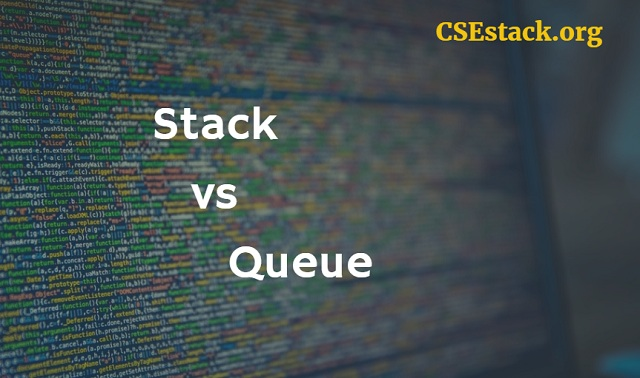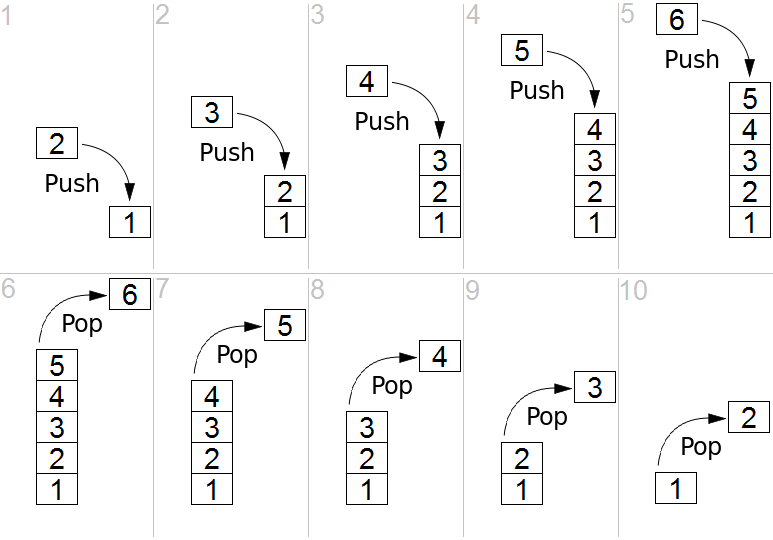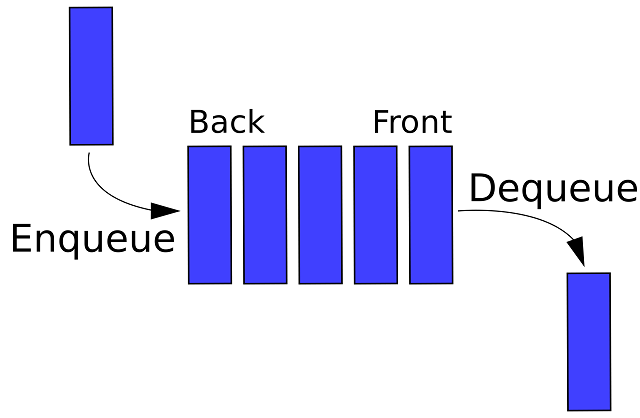# Difference Between Stack and Queue in Data Structure

Stack and Queue are the very important data structures in programming. Whether you are writing a complex program or preparing for placement or getting into the career, you will come across questions related to the basic difference between stack and queue.

With stack and queue data structures, it is very easy to solve even complex programming questions.

Here are the topics you are going to learn from this article.For the sake of simplicity, we can define stack and queue as the collections of the objects, just like an array in the data structure. This is the only similarity between stack and queue. But, they are not mean to be similar in any way.

#### What is a Stack?

The stack is a data structure where the user can add a data object any time. But, the user can only remove the data which is added at last.

The stack follows LIFO (Last In First Out) mechanism. It means the object which is added last only can be removed.

This is reverse in case of the queue.

#### What is a Queue?

The queue is a data structure where the user can add data object at any time. But the user can only remove the data which is added first.

Unlike stack, the queue follows FIFO (First In First Out) mechanism. It means an object which is added first only can be removed.

If you compare the stack and queue, you can see the difference is in order of removing element/object. The order of removal of data object defines the use of these two data structures.

Let’s see their uses with applications.

### Applications | When to Use  Stack vs Queue?

Learning these data structures does not make any sense if you don’t know where to use them. So, here are some examples.

Where is stack useful?

Use the stack for storing data elements, when you need recently added object to be treated/processed first.

Example: In the searching algorithm, one of the primary application of the stack is DFS (Depth-First Search).

Where should you use the queue?

In the programming, the queue is useful to store the data elements when you want to treat or process element which is added first.

The person enters a restaurant first gets service first.

Example: Queue is used in BFS (Breadth First Search)  algorithm.

Understanding Stack and Queue for Career point of view:

If you are preparing for GATE or any other competitive CS exam, you may be asked to find the valid sequence for the elements stored in stack or queue.

So understanding the difference between the operations of stack and queue is crucial.

## Difference Between Stack and Queue by Their Operations

What are the operations are involved in Stack?

Stack uses ‘top’ pointer which is always pointed to the most recently added element in the stack.#### PUSH Operation on Stack:

The operation of adding an element to the stack is PUSH operation.

Steps:

• Find the pointer pointing to the top element of the stack.
• Add an object to the top of the stack.
• Increment the pointer and point it to the newly added object.

#### POP Operation on Stack:

The operation of removing top elements from the stack is PUSH operation.

Steps:

• Check if the stack is not empty.
• Access the topmost object using the top pointer. (Save the object in a local variable to use in the application.)
• Remove the object from the top.
• Point the ‘top’ pointer to the next object in the stack.

Even it is possible to implement stack operation using an array.

What are the operations are involved in the Queue?

Unlike stack data structure, queue requires two pointers so-called FRONT and REAR.Here ‘Back’ pointer is the same as ‘Rear’ pointer. So don’t get confused.

#### Enqueue operation on Queue:

The operation of adding an element in the queue is callaed as Enqueue operation.

Steps:

• Find the REAR pointer of the queue.
• Increment the REAR pointer.
• Add new object at the REAR pointer.

In Enqueue operation you don’t need to do anything with the FRONT pointer.

### Dequeue operation on Queue:

The operation of removing an element from the queue is called as Dequeue operation.

Steps:

• Ensure if the queue is not empty.
• Remove the object. (Store in a local variable to use in the application.)
• Increment the FRONT pointer to point it to next front object.

In Dequeue operation you don’t need to do anything with the REAR pointer.

Operation Bound:

The stack is bounded from one end. There is only one end to carry both push and pop operation.

The queue is free from both sides. One can dequeue an element from one end and enqueue element from another end.

These operations are crucial to finding the difference between stack and queue.

### Memory Usage Comparison of Stack and Queue:

Both data structure are useful to store the data objects. So it will take almost the same size memory to store the same amount of data elements.

If you go down the further…

The stack needs only one pointer so-called top pointer. (It points to the topmost object in the stack.)

The queue requires two pointers. (One is FRONT and other is REAR pointer.)

#### Example of Stack and Queue

An example of a Stack:

When a program calls a subroutine, which calls another subroutine and so on… You need a stack to store these recursive subroutine calls.

So the subroutine execution occurs in reverse order (LIFO).

An example of a Queue:

Whenever the system receives interrupt call, the system adds it to the event queue. System services them in the same order as they appear.

So the interrupt calls are handled in FIFO manner.

### Difference Between Stack and Queue in Tabular Form

No. STACK QUEUE
1 Data insertion and data removing occur only at one end. Data insertion and data removing occur at two different ends.
2 Stack follows LIFO mechanism. Queue follows FIFO mechanism.
3 Adding operation in the stack is called as PUSH operation. Adding operation in the queue is called as Enqueue operation.
4 Removing element in the stack is called as POP operation. Removing element in the queue is called as Dequeue operation.
5 Stack require only one pointer so-called “top” pointer. Queue uses two pointers so-called “Front” and “Rear” pointers.
6 Example: The way recursive system call works, it uses the Stack mechanism. Example: A system interrupt is a good example where the queue mechanism is used.

#### How Array is different from Stack and Queue?

I know it’s not the part of this article. But it’s good for your understanding, how stack and queue are different from the array.

The array is a data structure where you can add or remove any object you wish. It is possible by assigning an index to each element of the array. You can call that object by its index. It’s pretty simple.

You can not access the stack/queue element by its index. In fact, they don’t have indexing.

Related Read: Difference between Min and Max Heap

### Final Thought over Stack Vs Queue:

Needless to say, stack and queue have similarity as they stores the collection of data objects. But they are entirely different for their mechanism to access, remove and store the data elements.

The stack is LIFO and Queue is FIFO data structure. Both are very useful in the context of writing a complex program. In actual programming, you have to be very clever to understand the difference between stack and queue, whether you need to use the stack or queue in your program.

1.harshni says:

It was one of the great explanation which I have seen….

1.Aniruddha Chaudhari says:

Thanks, Harshni!

Glad you like it. I will try my best to stick with more of such content. Stay tuned!

2.Deep Raj says:

Thank you for this post or article I have confused with many concepts in DS this helped me a lot. Once again thank you!

1.Aniruddha Chaudhari says:

You’re welcome, Deep!